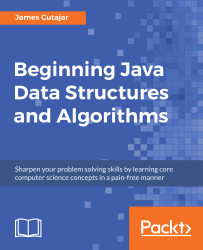•#### Beginning Java Data Structures and Algorithms#### Overview of this book

Learning about data structures and algorithms gives you a better insight on how to solve common programming problems. Most of the problems faced everyday by programmers have been solved, tried, and tested. By knowing how these solutions work, you can ensure that you choose the right tool when you face these problems. This book teaches you tools that you can use to build efficient applications. It starts with an introduction to algorithms and big O notation, later explains bubble, merge, quicksort, and other popular programming patterns. You’ll also learn about data structures such as binary trees, hash tables, and graphs. The book progresses to advanced concepts, such as algorithm design paradigms and graph theory. By the end of the book, you will know how to correctly implement common algorithms and data structures within your applications.
Title PagePackt UpsellContributorsPrefaceFree Chapter
Algorithms and ComplexitiesSorting Algorithms and Fundamental Data StructuresHash Tables and Binary Search TreesString Matching AlgorithmsGraphs, Prime Numbers, and Complexity ClassesOther Books You May EnjoyIndex## Understanding Complexity Classes of Problems

Nearly all of the algorithms introduced so far run in polynomial time (for instance, on inputs of size n, their worst-case running time is O(nk) for constant k). However, there are problems that simply cannot be solved or for which a polynomial-time algorithm hasn't been found yet.

An example of a problem that cannot be solved is the halting problem. The halting problem is the problem of determining, from the description of a computer program and an input, whether the program will finish running or continue to run forever. Alan Turing proved that a general algorithm to solve the halting problem for all pairs of (program, input) cannot exist.

It is common to call problems solvable by polynomial-time algorithms (for instance, those whose worst-case running time is O(nk) for constant k) as "tractable", or "easy", and problems that require a super-polynomial-time algorithm (for instance, those whose running time is not bounded above by any polynomial...